Ex 9.1

Chapter 9 Class 10 Some Applications of Trigonometry (Term 2)
Serial order wise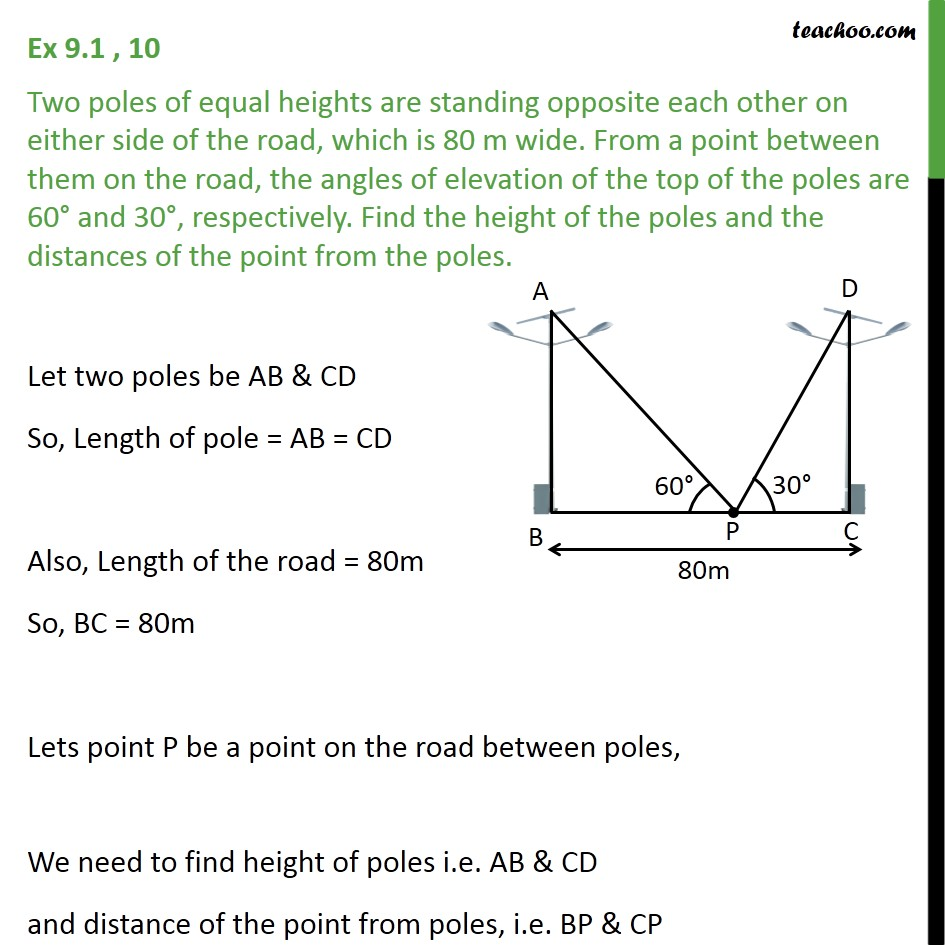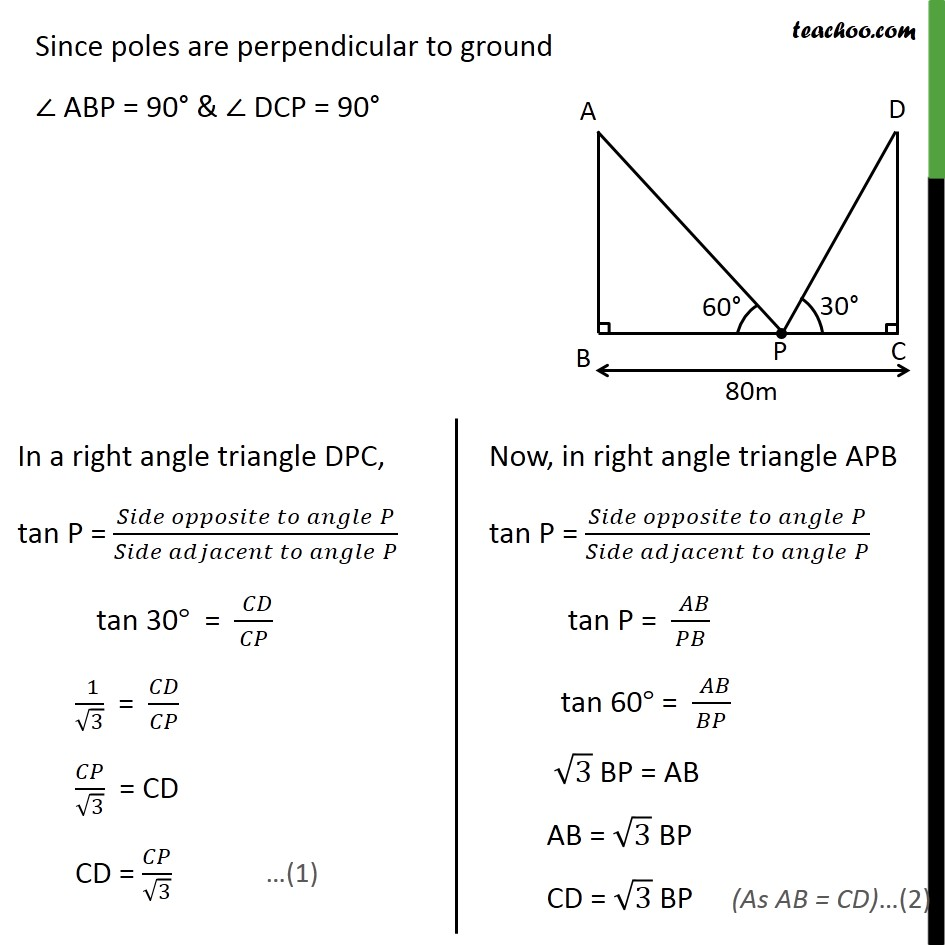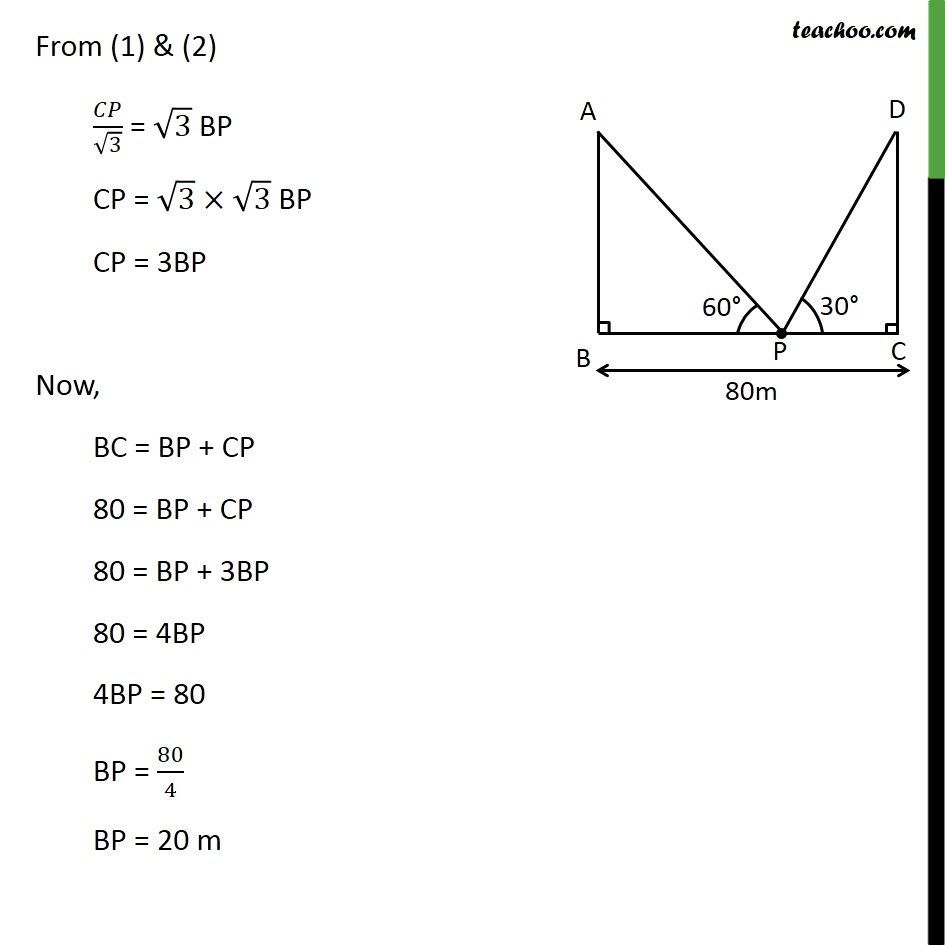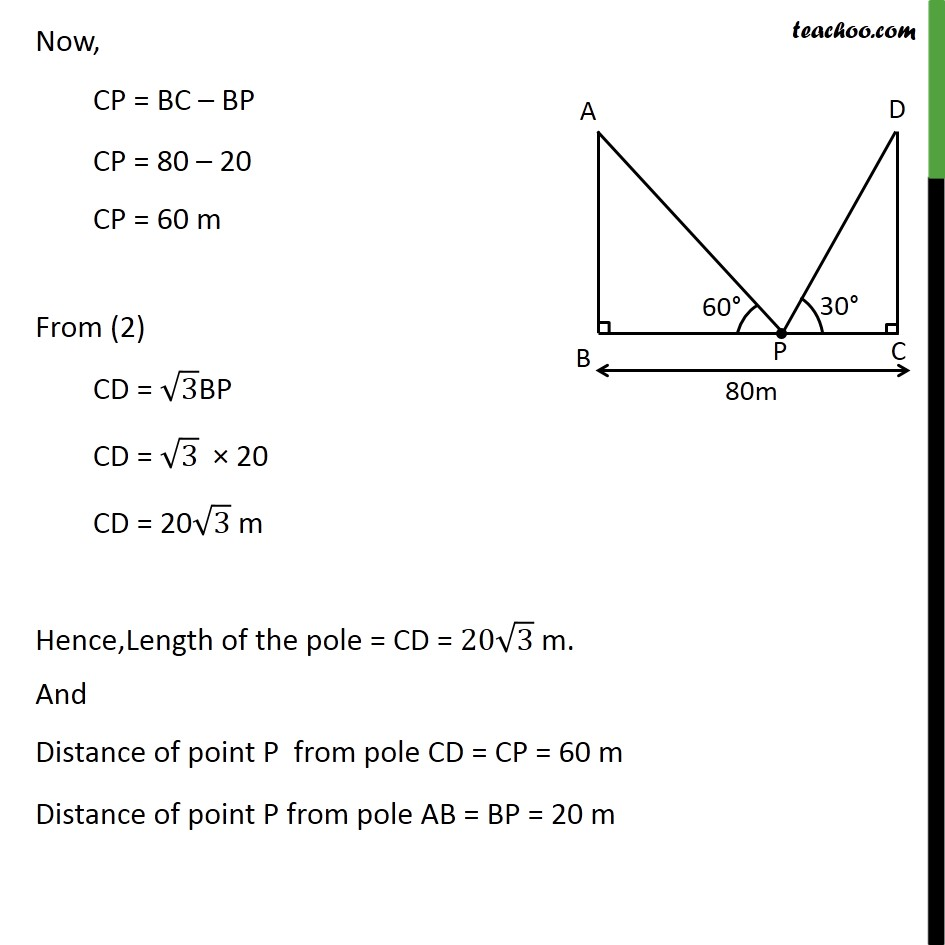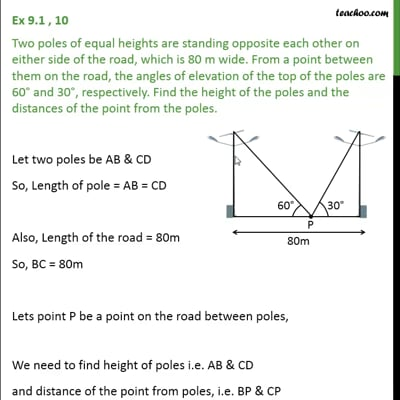This video is only available for Teachoo black users

### Transcript

Ex 9.1 , 10 Two poles of equal heights are standing opposite each other on either side of the road, which is 80 m wide. From a point between them on the road, the angles of elevation of the top of the poles are 60° and 30°, respectively. Find the height of the poles and the distances of the point from the poles. Let two poles be AB & CD So, Length of pole = AB = CD Also, Length of the road = 80m So, BC = 80m Lets point P be a point on the road between poles, We need to find height of poles i.e. AB & CD and distance of the point from poles, i.e. BP & CP Since poles are perpendicular to ground ∠ ABP = 90° & ∠ DCP = 90° From (1) & (2) 𝐶𝑃/√3 = √3 BP CP = √3×√3 BP CP = 3BP Now, BC = BP + CP 80 = BP + CP 80 = BP + 3BP 80 = 4BP 4BP = 80 BP = 80/4 BP = 20 m Now, CP = BC – BP CP = 80 – 20 CP = 60 m From (2) CD = √3BP CD = √3 × 20 CD = 20√3 m Hence,Length of the pole = CD = 20√3 m. And Distance of point P from pole CD = CP = 60 m Distance of point P from pole AB = BP = 20 m